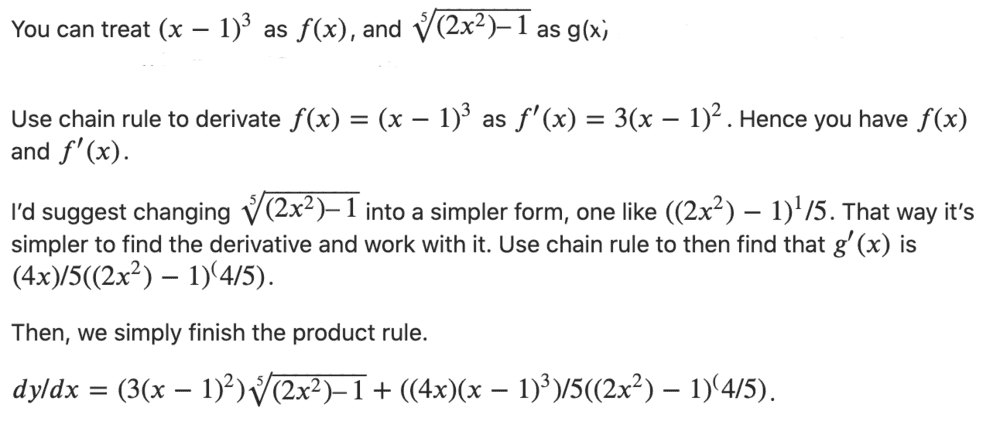# Differentiating y = (x -1)^3 (5√2x^2 -1)

Homework Statement:
I'm supposed to differentiate, but I was asked not to simplify..I'm not sure if my final answer is formatted such that it can be understood...
Relevant Equations:
n/a$$\frac{dy}{dx} = 3(x - 1)^2 (2x^2 - 1)^{1/5} + \frac{4x(x - 1)^3}{5(2x^2 - 1)^{4/5}}$$